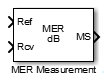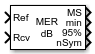# MER Measurement

Measure modulation error ratio in digital modulation applications

• Library:
• Communications Toolbox / Utility Blocks

•## Description

The MER Measurement block computes a form of signal-to-noise ratio (SNR) measurement that you can use to assess the ability of a receiver to accurately demodulate a signal. Specifically, it returns the modulation error ratio (MER), minimum MER, and percentile MER for a received signal. You use the MER measurements to determine system performance in communications applications. For example, to determine compliance with applicable DVB-T system radio transmission standards conformance testing requires accurate MER measurements.

This icon shows the block with all ports enabled.## Ports

### Input

expand all

Reference signal, specified as a scalar, vector, matrix, or 3-D array. If you specify this input, the object measures the MER of the `Rcv` input by using this input as a reference constellation.

The dimensions of this input must match those of the `Rcv` input. The object uses each element of this input as the reference symbol for the corresponding element of the `Rcv` input.

#### Dependencies

To enable this port, set the Reference signal parameter to `Input port`.

Data Types: `single` | `double` | `fixed point`
Complex Number Support: Yes

Received signal, specified as a scalar, vector, matrix, or 3-D array.

Data Types: `single` | `double` | `fixed point`
Complex Number Support: Yes

### Output

expand all

Percentage MER of the received signal over the configured measurement interval, returned as a scalar in units of decibels.

Data Types: `double`

Minimum percentage MER over the configured measurement interval, returned as a scalar in units of decibels.

#### Dependencies

To enable this port, select the Output minimum MER parameter.

Data Types: `double`

Value below which X% of MER measurements fall since the last reset, returned as a scalar in units of decibels. Set the value of X in the X-percentile value (%) parameter.

#### Dependencies

To enable this port, select the Output X-percentile MER parameter.

Data Types: `double`

Number of symbols that the object uses to measure the output, returned as a positive integer.

#### Dependencies

To enable this port, select the Output X-percentile MER and Output the number of symbols processed parameters.

Data Types: `double`

## Parameters

expand all

Reference signal source, specified as ```Input port``` or ```Estimated from reference constellation```.

Reference constellation points, specified as a vector.

#### Dependencies

To enable this parameter, set the Reference signal parameter to ```Estimated from reference constellation```.

Data Types: `double`
Complex Number Support: Yes

Measurement interval source for MER and minimum MER measurements, specified as one of these values.

• `Input length` — Measure the MER using only the current samples.

• `Entire history` — Measure the MER for all samples.

• `Custom` — Measure the MER by using a sliding window over an interval that you specify.

• `Custom with periodic reset` — Measure the MER over an interval that you specify and reset the block after measuring over each interval.

Custom measurement interval in samples, specified as a positive integer.

#### Dependencies

To enable this parameter, set the Measurement interval parameter to `Custom` or `Custom with periodic reset`.

Data Types: `double`

Dimensions over which the block averages the MER measurements, specified as an integer or row vector of integers in the range [1, 3]. For example, to average across the columns, set this parameter to `2`.

This block supports variable-size inputs of the dimensions across which the averaging takes place. However, the input size for the nonaveraged dimensions must remain constant. For example, if the input has size ```[1000 3 2]``` and you set this parameter to `[1 3]`, then the output size is `[1 3 1]` and the number of elements in the second dimension must remain fixed at `3`.

Data Types: `double`

Option to add the `min` port to output minimum MER measurements. To enable minimum MER measurements, select this parameter.

Option to add the `X%` port to output X-percentile MER measurements. To enable X-percentile MER measurements, select this parameter. When you select this parameter, X-percentile MER measurements persist until you reset the block. The block performs these measurements by using all of the input frames since the last reset. You can set the value of X in the X-percentile value (%) parameter.

Value below which X% of MER measurements fall, specified as a scalar in the range [0, 100].

#### Dependencies

To enable this parameter, select the Output X-percentile MER parameter.

Data Types: `double`

Option to add the `nSym` port to output the number of symbols used to measure the X-percentile MER. To measure the number of symbols used for X-percentile MER measurements, select this parameter.

#### Dependencies

To enable this parameter, select the Output X-percentile MER parameter.

Type of simulation to run, specified as ```Interpreted execution``` or `Code generation`.

• `Interpreted execution` — Simulate the model by using the MATLAB® interpreter. This option requires less startup time than the `Code generation` option, but the speed of subsequent simulations is slower. In this mode, you can debug the source code of the block.

• `Code generation` — Simulate the model by using generated C code. The first time you run a simulation, Simulink® generates C code for the block. The C code is reused for subsequent simulations unless the model changes. This option requires additional startup time, but the speed of the subsequent simulations is faster than `Interpreted execution`.

## Block Characteristics

 Data Types `double` | `fixed point` | `integer` | `single` Multidimensional Signals `no` Variable-Size Signals `no`

## Algorithms

MER is a measure of the SNR in a modulated signal calculated in dB. The MER over a burst containing N symbols is

`$MER=10×\text{​}{\mathrm{log}}_{10}\left(\frac{\sum _{k=1}^{N}\left({I}_{k}^{2}+{Q}_{k}^{2}\right)}{\sum _{k=1}^{N}\left({e}_{k}\right)}\right)\text{\hspace{0.17em}}\text{dB,}$`

where:

• ek = ${e}_{k}={\left({I}_{k}-{\stackrel{˜}{I}}_{k}\right)}^{2}+{\left({Q}_{k}-{\stackrel{˜}{Q}}_{k}\right)}^{2}$.

• Ik represents the in-phase component of the kth symbol in the burst.

• Qk represents the quadrature phase component of the kth symbol in the burst.

• Ik and Qk represent ideal reference values.

• ${\stackrel{˜}{I}}_{k}$ and ${\stackrel{˜}{Q}}_{k}$ represent received symbols.

• N represents the number of symbols in the burst.

The MER for the kth symbol is

`$ME{R}_{k}=10×\text{​}{\mathrm{log}}_{10}\left(\frac{\frac{1}{N}\sum _{k=1}^{N}\left({I}_{k}^{2}+{Q}_{k}^{2}\right)}{{e}_{k}}\right)\text{\hspace{0.17em}}\text{dB}\text{.}$`

The minimum MER represents the minimum MER value in a burst, or

$ME{R}_{\mathrm{min}}=\underset{k\in \left[1,...,N\right]}{\mathrm{min}}\left\{ME{R}_{k}\right\}\text{\hspace{0.17em}},$

The algorithm computes the X-percentile MER by creating a histogram of all the incoming MERk values. The output provides the MER value above which X% of the MER values fall.

 ESTI TR 101 290. Digital Video Broadcasting (DVB): Measurement guidelines for DVB systems. June 2020.